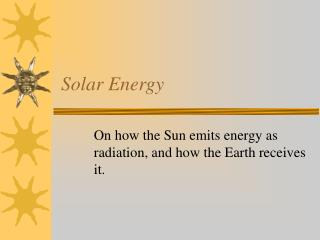DownloadDownload PresentationSolar Energy

# Solar Energy

Download Presentation## Solar Energy

- - - - - - - - - - - - - - - - - - - - - - - - - - - E N D - - - - - - - - - - - - - - - - - - - - - - - - - - -
##### Presentation Transcript

1. Solar Energy On how the Sun emits energy as radiation, and how the Earth receives it.

2. Outline • Blackbody radiation and the Sun. • Wien’s law • Stefan-Boltzmann law • The temperature and spectrum from the Sun. • The Earth as receiver • The Solar Constant • The wobbly Earth • The Atmospheric Filter and albedo • The absorbing/reflecting surface • Blackbody re-emission.

3. Blackbody Spectrum and Temperature

4. Wien’s law • A blackbody emits the greatest percentage of its energy at a single wavelength, called λmax. • That wavelength depends on the blackbody’s temperature, according to Wien’s law. • λmax = .0029 m*K / T(K) • Example: • If T = 500 K, λmax = .0029 m*K / T(K) = 5.8 x 10-6m • Looking at our EM chart, that is in the Infrared.

5. The Sun’s spectrum

6. The Sun’s temperature • From the graph, • λmax = .5 m or 5 x 10-7 m or 5000 . • Then Wien’s law says: • λmax = .0029 m*K / T(K) or • T(K) = .0029 m*K / 5 x 10-7 m = 5,800 K • The temperature of the Sun’s surface is 5,800 K! • It’s spectrum is centered in the Visible range (44%), with a long tail in the Infrared, and a significant amount of Ultraviolet, as well.

7. Stefan-Boltzmann Law • The power (energy per time) per area emitted by a blackbody is also a function of Temperature. • Stefan-Boltzmann law: • Power / Area =  T4 •  = 5.67 x 10-8 W/m2*K4 • Example: Our body temperature is T = 310 K • If we were a blackbody, we would emit 500 W per square meter of area. Actually, we emit far less because we are not close to being a blackbody. We end up emitting around 1,000 food calories in a day.

8. Power radiated by the Sun • T sun = 5800 K • P/A = Power / Area =  T4 • = 64 MW / m2 • The Sun emits enough energy per square meter to supply all the energy needs of a small city!

9. But what do we get out of it? • Solar constant = 1360 W/m^2. • 93 million miles away! • Earth’s tilt! • Atmospheric absorption.

10. Earth’s tilt

11. Atmospheric absorption